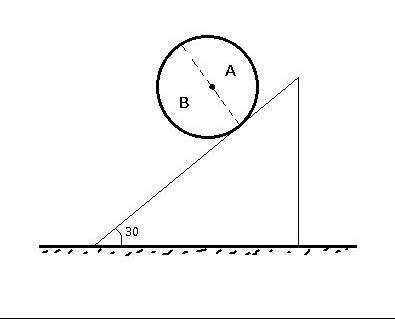# Sir plzz help.....A ring as shown in the 'figure' is made up of two semi circular rings A and B of masses " 2 Kg " and " 4 Kg " respectively. The ring has diameter " 1 m ". If the Ring rolls without slipping, Find –
1.Angular Acceleration
2.Normal Reaction and
3. Frictional force

•Anurag Ghosh ·

In this question would we have to account for the force exerted by the one semi-circular ring on the other????

•Akash Anand ·

The masses of two rings are different then the only change will be in the moment of inertia (I) part. Remaining part going to be the same.

And if the two parts are exerting any force on one another, then why we should bother about that? Do we need that?

•